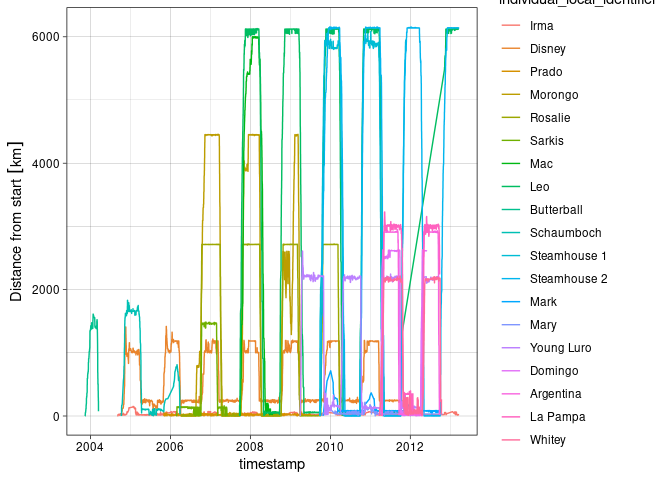# Trajectory analysis of Turkey vultures

## Retrieve data

library(move2)
vulture_data <-
vulture_data
#> A <move2> with track_id_column "individual_local_identifier" and
#> time_column "timestamp"
#> Containing 19 tracks lasting on average 1083 days in a
#> Simple feature collection with 215719 features and 6 fields
#> Geometry type: POINT
#> Dimension:     XY
#> Bounding box:  xmin: -123.9255 ymin: -39.8214 xmax: -48.5788 ymax: 54.01817
#> Geodetic CRS:  WGS 84
#> # A tibble: 215,719 × 7
#>   sensor_type_id individual_local_identifier manually_marked_outlier
#>          <int64> <fct>                       <lgl>
#> 1            653 Irma                        FALSE
#> 2            653 Irma                        FALSE
#> 3            653 Irma                        FALSE
#> 4            653 Irma                        FALSE
#> 5            653 Irma                        FALSE
#> # ℹ 215,714 more rows
#> # ℹ 4 more variables: timestamp <dttm>, event_id <int64>, visible <lgl>,
#> #   geometry <POINT [°]>
#> First 5 track features:
#> # A tibble: 19 × 54
#>   deployment_id   tag_id individual_id animal_life_stage animal_mass
#>         <int64>  <int64>       <int64> <fct>                     [g]
#> 1      17225120 16883951      17002744 adult                    2372
#> 2      17225134 16883951      17002745 adult                    1951
#> 3      17225131 16883928      17002732 adult                    2012
#> 4      17225133 16883937      17002737 adult                    2108
#> 5      17225122 16883967      17002753 adult                      NA
#> # ℹ 14 more rows
#> # ℹ 49 more variables: attachment_type <fct>, deployment_comments <chr>,
#> #   deploy_off_timestamp <dttm>, deploy_on_timestamp <dttm>, duty_cycle <chr>,
#> #   deployment_local_identifier <fct>, study_site <chr>, sensor_type_ids <chr>,
#> #   capture_location <POINT [°]>, deploy_on_location <POINT [°]>,
#> #   deploy_off_location <POINT [°]>, individual_comments <chr>,
#> #   individual_local_identifier <fct>, sex <fct>, taxon_canonical_name <fct>, …

In this case some tracks have very long time gaps, to prevent distant points to be connected by a line we split those tracks by creating a new id.

library(dplyr, quietly = TRUE)
library(ggplot2, quietly = TRUE)
library(rnaturalearth, quietly = TRUE)
#> Support for Spatial objects (sp) will be deprecated in {rnaturalearth} and will be removed in a future release of the package. Please use sf objects with {rnaturalearth}. For example: ne_download(returnclass = 'sf')
library(units, quietly = TRUE)
vulture_lines <- vulture_data %>%
mutate_track_data(name = individual_local_identifier) %>%
mutate(
large_gaps = !(mt_time_lags(.) < set_units(1500, "h") |
is.na(mt_time_lags(.))),
track_sub_id = cumsum(lag(large_gaps, default = FALSE)),
new_track_id = paste(mt_track_id(.), track_sub_id)
) %>%
mt_set_track_id("new_track_id") %>%
mt_track_lines()
#> Joining with by = join_by(new_track_id)
ggplot() +
geom_sf(data = ne_coastline(returnclass = "sf", 50)) +
theme_linedraw() +
geom_sf(
data = vulture_lines,
aes(color = name)
) +
coord_sf(
crs = sf::st_crs("+proj=aeqd +lon_0=-83 +lat_0=8 +units=km"),
xlim = c(-3500, 3800), ylim = c(-4980, 4900)
)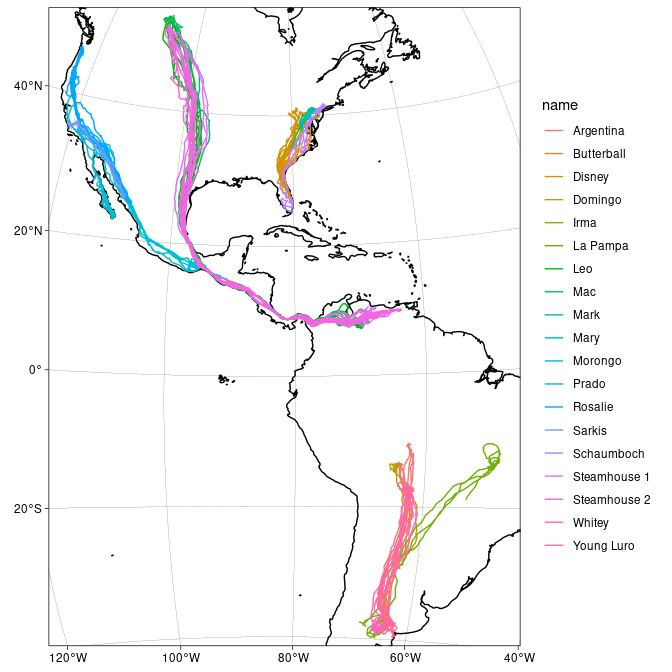## Categorize seasons

library(magrittr, quietly = TRUE)
library(lubridate, quietly = TRUE)
vulture_data %<>% mutate(
month = month(mt_time(), label = TRUE, abbr = FALSE),
season = recode_factor(month,
January = "Wintering", February = "Wintering",
March = "Wintering", April = "North migration",
May = "North migration", June = "Breeding",
July = "Breeding", August = "Breeding",
September = "South migration", October = "South migration",
November = "Wintering", December = "Wintering"
),
season = if_else(season == lead(season, 1) &
season, NA
)
)

Annotate speed and azimuth to the trajectory.

vulture_data %<>% mutate(azimuth = mt_azimuth(.), speed = mt_speed(.))

## Seasonal distribution per individual

library(circular, quietly = TRUE)
#>
#> Attaching package: 'circular'
#> The following objects are masked from 'package:stats':
#>
#>     sd, var
vulture_azimuth_distributions <- vulture_data %>%
filter(speed > set_units(2, "m/s") & !is.na(season)) %>%
group_by(season, track_id = mt_track_id()) %>%
filter(n() > 50) %>%
summarise(azimuth_distribution = list(density(
as.circular(
drop_units(set_units(
azimuth,
"degrees"
)),
units = "degrees",
modulo = "asis",
zero = 0,
template = "geographic", rotation = "clock", type = "angles"
),
bw = 180, kernel = "vonmises"
)))
#> summarise() has grouped output by 'season'. You can override using the
#> .groups argument.

# Load purrr for map function
library(purrr, quietly = TRUE)
#>
#> Attaching package: 'purrr'
#> The following object is masked from 'package:magrittr':
#>
#>     set_names
# Load tidy r for unnest function
library(tidyr, quietly = TRUE)
#>
#> Attaching package: 'tidyr'
#> The following object is masked from 'package:magrittr':
#>
#>     extract
vulture_azimuth_distributions %>%
mutate(
x = map(azimuth_distribution, ~ .$x), y = map(azimuth_distribution, ~ .$y)
) %>%
select(-azimuth_distribution) %>%
unnest(c(x, y)) %>%
ggplot() +
geom_path(aes(x = x, y = y, color = season)) +
coord_polar(start = pi / 2) +
theme_linedraw() +
facet_wrap(~ factor(track_id)) +
scale_x_continuous(
name = NULL, breaks = (-2:1) * 90,
labels = c("S", "W", "N", "E")
) +
scale_y_continuous(name = NULL, limits = c(-0.8, 1.0), expand = c(0L, 0L))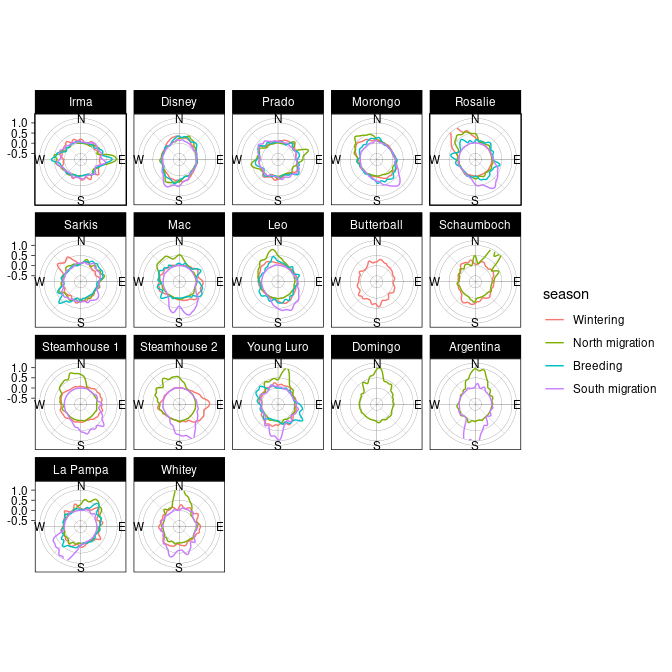## Individual trajectory

leo <- vulture_data |>
filter_track_data(individual_local_identifier == "Leo") |>
mutate(speed_categorical = cut(speed, breaks = c(2, 5, 10, 15, 35)))
leo |> ggplot(aes(x = azimuth, y = speed)) +
geom_point() +
scale_x_units(unit = "degrees", breaks = c(-2:2) * 90, expand = c(0L, 0L)) +
theme_linedraw()
#> Warning: Removed 6683 rows containing missing values (geom_point()).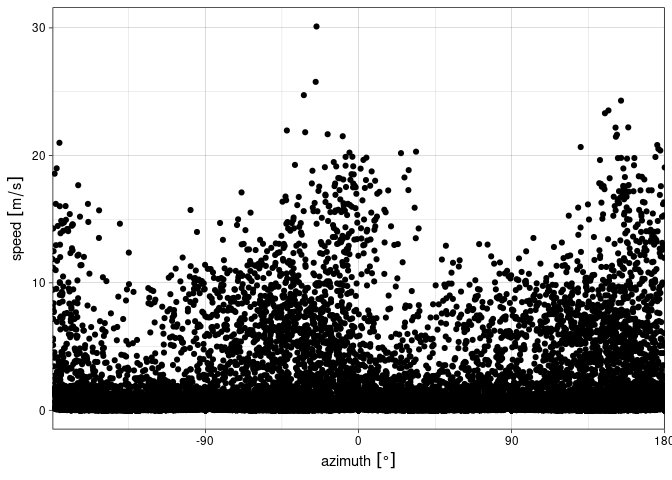leo |>
filter(speed > set_units(2L, "m/s") & !is.na(season)) |>
ggplot() +
coord_polar(start = pi) +
geom_histogram(
aes(
x = set_units(azimuth, "degrees"),
fill = speed_categorical
),
breaks = set_units(seq(-180L, 180L, by = 10L), "degrees"),
position = position_stack(reverse = TRUE)
) +
scale_x_units(
name = NULL,
limits = set_units(c(-180L, 180L), "degrees"),
breaks = (-2L:2L) * 90L
) +
facet_wrap(~season) +
scale_fill_ordinal("Speed") +
theme_linedraw()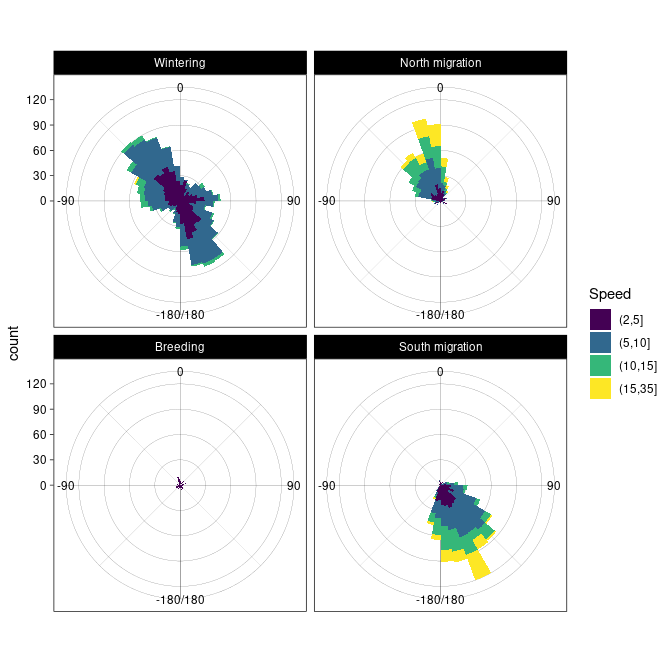Plot turn angle distribution.

pi_r <- set_units(pi, "rad")
leo %>%
mutate(turnangle = mt_turnangle(.)) %>%
filter(speed > set_units(2L, "m/s") & lag(speed, 1L) > set_units(2L, "m/s")) %>%
ggplot() +
geom_histogram(
aes(
x = turnangle,
fill = speed_categorical
),
position = position_stack(reverse = TRUE)
) +
scale_fill_ordinal("Speed") +
coord_polar(start = pi) +
scale_x_units(limits = c(-pi_r, pi_r), name = NULL) +
scale_y_continuous(limits = c(-500L, 650L), breaks = c(0L, 250L, 500L)) +
theme_linedraw()
#> stat_bin() using bins = 30. Pick better value with binwidth.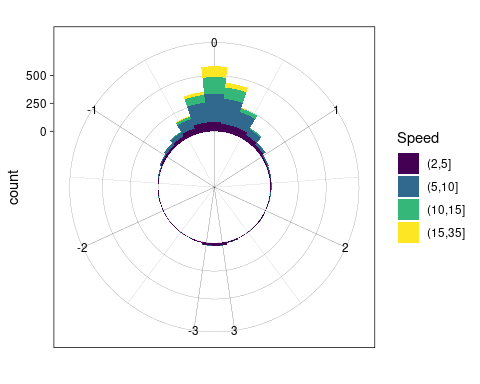## Net displacement

Here we visualize the distance to the first location of each trajectory.

vulture_data %<>% group_by(mt_track_id()) %>%
mutate(displacement = c(st_distance(
!!!syms(attr(., "sf_column")),
(!!!syms(attr(., "sf_column")))[row_number() == 1]
)))

vulture_data %>% ggplot() +
geom_line(aes(
x = timestamp,
y = set_units(displacement, "km"),
color = individual_local_identifier
)) +
ylab("Distance from start") +
theme_linedraw()